The most widely used approach and also what the WinTess3 program provides is called Force density within the scope of simulation method (as commented in the introduction).

This method is based on studying a mesh that simulates a membrane which is in equilibrium when subjected to forces. Given that the mesh is not a continuous surface, but a set of bars, the forces applied to the mesh are assumed applied to the nodes, as shown in the following figure.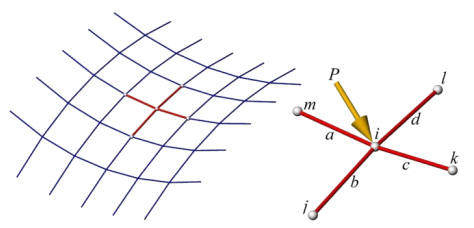Where a, b, c and d are the bars that meet at the point i on which we have an applied force of P.

These bars a, b, c and d are subject to forces Sa, Sb, Sc, and Sd. These forces are in the 3D space, therefore we can decompose them into its three components according to the axes X,Y,Z by multiplying the absolute value of the force by the cosine of the angle that forms between the bar and each of the three axes X,Y,Z.

If the mesh is in balance, the following must be met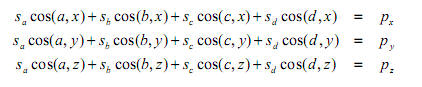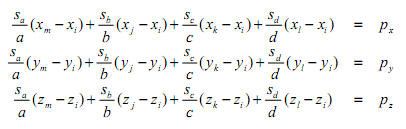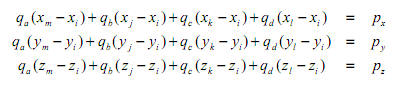In these equations, the lengths a, b, c and d are non-linear functions of the coordinates. In addition, the forces are dependent on the unstressed mesh widths and on Hooke’s law. Substituting these functions would lead to a finite element formulation.

Based on the interaction of form and forces, the form-finding process aims to obtain the geometry of a form with a desired prestress and a surface discretisation of a desired mesh.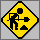Text is based on a presentation entitled: “A HISTORY OF THE MAIN DEVELOPMENTS AND APPLICATIONS OF THE FORCE DENSITY METHOD IN GERMANY 1970-1999”, the IASS-IACM 2000, Fourth International Colloquium on Computation of Shell & Spatial Structures, by the authors Lothar Gründig Erik Moncrieff, Peter Singer and Dieter Ströbel.